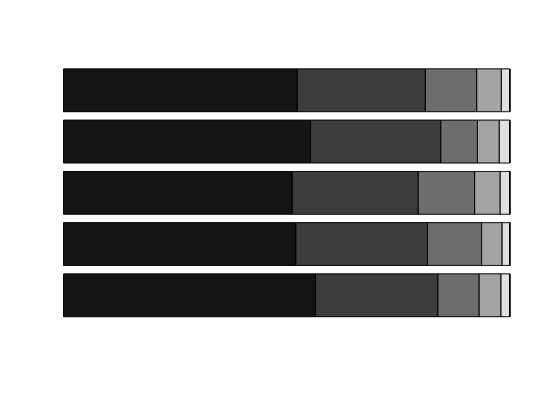This function creates a descriptive plot for a question in an endorsement experiment.

endorse.plot(Y, data, scale, dk = 98, ra = 99, yaxis = NULL,
col.seq = NA)

## Arguments

Y a character vector. List of the variable names for the responses to a question. Each variable name corresponds to each treatment status. data frame containing the variables. an integer. The scale of the responses. The function assumes that the responses are coded so that 1 indicates the lowest support while the integer specified in this argument represents the highest support. an integer indicating the value of the response variable that is to be interpreted as Don't Know.'' Default is 98. an integer indicating the value of the response variable that is to be interpreted as Refused.'' Default is 99. a character vector of the same length as Y. The argument will be used for the label of the horizontal axis. The order should be the same as Y. a vector of colors for the bars or bar components. By default, a gradation of gray where the darkest indicates the highest support level.

## Value

A descriptive plot for the responses to a question.

## Examples


data(pakistan)

Y <- c("Polio.a", "Polio.b", "Polio.c", "Polio.d", "Polio.e")
yaxis <- c("Control", "Kashmir", "Afghan", "Al-Qaida", "Tanzeems")

endorse.plot(Y = Y, data = pakistan, scale = 5)